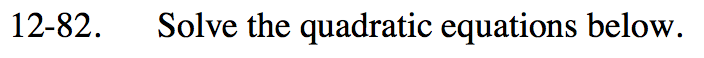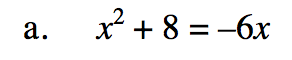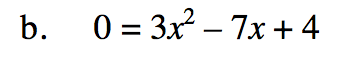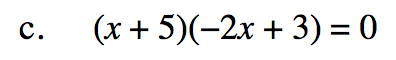### Home > INT2 > Chapter 12 > Lesson 12.2.2 > Problem12-82

12-82.

Solve the quadratic equations below. Homework Help ✎

 a. x2 + 8 = –6x b. 0 = 3x2 – 7x + 4 c. (x + 5)(−2x + 3) = 0Use factoring and zero product property or the quadratic formula.

x = 1; x = 4/3